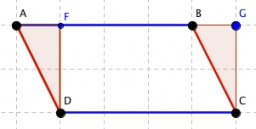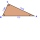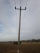# Parallelogram

Find the parallelogram's perimeter, where base a = 8 cm, height v = 3 cm, and angle alpha = 35° is the magnitude of the angle at vertex A.

o =  26.4607 cm

### Step-by-step explanation:Did you find an error or inaccuracy? Feel free to write us. Thank you!Tips to related online calculators

#### You need to know the following knowledge to solve this word math problem:

We encourage you to watch this tutorial video on this math problem:

## Related math problems and questions:

• Diagonal BDFind the length of the diagonal BD in a rectangular trapezoid ABCD with a right angle at vertex A when/AD / = 8,1 cm and the angle DBA is 42°
• TrapezoidsIn the isosceles trapezoid ABCD we know: AB||CD, |CD| = c = 8 cm, height h = 7 cm, |∠CAB| = 35°. Find the area of the trapezoid.
• Parallelogram +ľ| AB | = 76cm, | BC | = 44cm, angle BAD = 30 °. Find the area of the parallelogram.
• A trapezoidA trapezoid with a base length of a = 36.6 cm, with angles α = 60°, β = 48° and the height of the trapezoid is 20 cm. Calculate the lengths of the other sides of the trapezoid.
• RectangularRectangular triangle KLM with right angle at vertex L, angle beta at vertex K and angle alpha at vertex M. Angle at vertex M = 65°, side l = 17.5 cm. Use Pythagorean theorems and trigonometric functions to calculate the lengths of all sides and the angle
• Parallelogram ABCDWe have the parallelogram ABCD, where AB is 6.2 cm BC is 5.4 cm AC is 4.8 cm calculate the height on the AB side and the angle DAB
• Ratio in trapeziumThe height v and the base a, c in the trapezoid ABCD are in the ratio 1: 6: 3, its content S = 324 square cm. Peak angle B = 35 degrees. Determine the perimeter of the trapezoid
• CalculateCalculate the area of triangle ABC, if given by alpha = 49°, beta = 31°, and the height on the c side is 9cm.
• Right triangle trigonometricsCalculate the size of the remaining sides and angles of a right triangle ABC if it is given: b = 10 cm; c = 20 cm; angle alpha = 60° and the angle beta = 30° (use the Pythagorean theorem and functions sine, cosine, tangent, cotangent)
• Right angleIn a right triangle ABC with a right angle at the apex C, we know the side length AB = 24 cm and the angle at the vertex B = 71°. Calculate the length of the legs of the triangle.In a regular quadrilateral pyramid, the height is 6.5 cm and the angle between the base and the side wall is 42°. Calculate the surface area and volume of the body. Round calculations to 1 decimal place.Find the area of a parallelogram if the diagonals u1 = 15 cm, u2 = 12 cm and the angle formed by them is 30 degrees.The outer angle of the triangle ABC at the A vertex is 71°40 ' outer angle at the vertex B is 136°50'. What size has the inner triangle angle at the vertex C?The height of a regular quadrilateral prism is v = 10 cm, the deviation of the body diagonal from the base is 60°. Determine the length of the base edges, the surface, and the volume of the prism.The magnitude of the internal angle at the main vertex C of the isosceles triangle ABC is 72°. The line p, parallel to the base of this triangle, divides the triangle into a trapezoid and a smaller triangle. How big are the inner angles of the trapezoid?The bases of two adjacent telegraph poles have a height difference of 10.5 m. How long do the wires connect the two poles if the slope is 39° 30´?The isosceles trapezoid ABCD has bases of 18 cm and 12 cm. The angle at apex A is 60°. What is the circumference and content of the trapezoid?